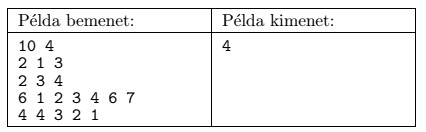Mathematical and Physical Journal
for High Schools
Issued by the MATFUND Foundation
 Already signed up? New to KöMaL?

#Problem S. 98. (April 2015)

S. 98. We are upgrading our computer, so we have to buy some components, and replace the old ones with new. We want to minimize the total cost, hence we should buy the least number of new components. Each component has the same price. However, it can happen that certain $\displaystyle k-1$ components out of a group of $\displaystyle k$ components belonging together have to be replaced. In this case the $\displaystyle k$th component should also be replaced. The shop contains $\displaystyle N$ types of components ($\displaystyle 1\le N\le 1\;000\;000$, numbered from $\displaystyle 1$ to $\displaystyle N$). A group of components belonging together can have an arbitrary size, but the total size of such groups is at most 250000. We also know that Component 1 should be replaced anyway.

Your program should read the values of $\displaystyle N$ and $\displaystyle C$ (the number of groups) from the first line of the standard input. Then, from each of the following $\displaystyle C$ lines, it should read the number of components $\displaystyle \mathrm{db}_i$ belonging to that group, finally the type of each component in that group as $\displaystyle \mathrm{db}_i$ integers between $\displaystyle 1$ and $\displaystyle N$. It is possible that two groups have some common components. Your program should write the minimal number of new components to be bought in the first line of the standard output.

In the example, Példa bemenet'' is a sample input, while Példa kimenet'' is the corresponding output. In this situation we have to replace Components 1, 2, 3 and 4.Scoring and bounds. You can obtain 1 point for a brief and proper documentation clearly describing your solution. Nine further points can be obtained provided that your program solves any arbitrary valid input within 1 second of running time.

The source code (s98.pas, s98.cpp, ...) without the .exe or any other auxiliary files generated by the compiler but with a short documentation (s98.txt, s98.pdf, ...), also describing which developer environment to use for compiling the source, should be submitted in a compressed file s98.zip.

(10 pont)

Deadline expired on May 11, 2015.

### Statistics:

 12 students sent a solution. 10 points: Alexy Marcell, Bálint Martin, Dóczi András, Gáspár Attila, Kiss Gergely, Molnár-Sáska Zoltán, Szakály Marcell, Weisz Ambrus. 9 points: Zalavári Márton. 8 points: 3 students.

Problems in Information Technology of KöMaL, April 2015